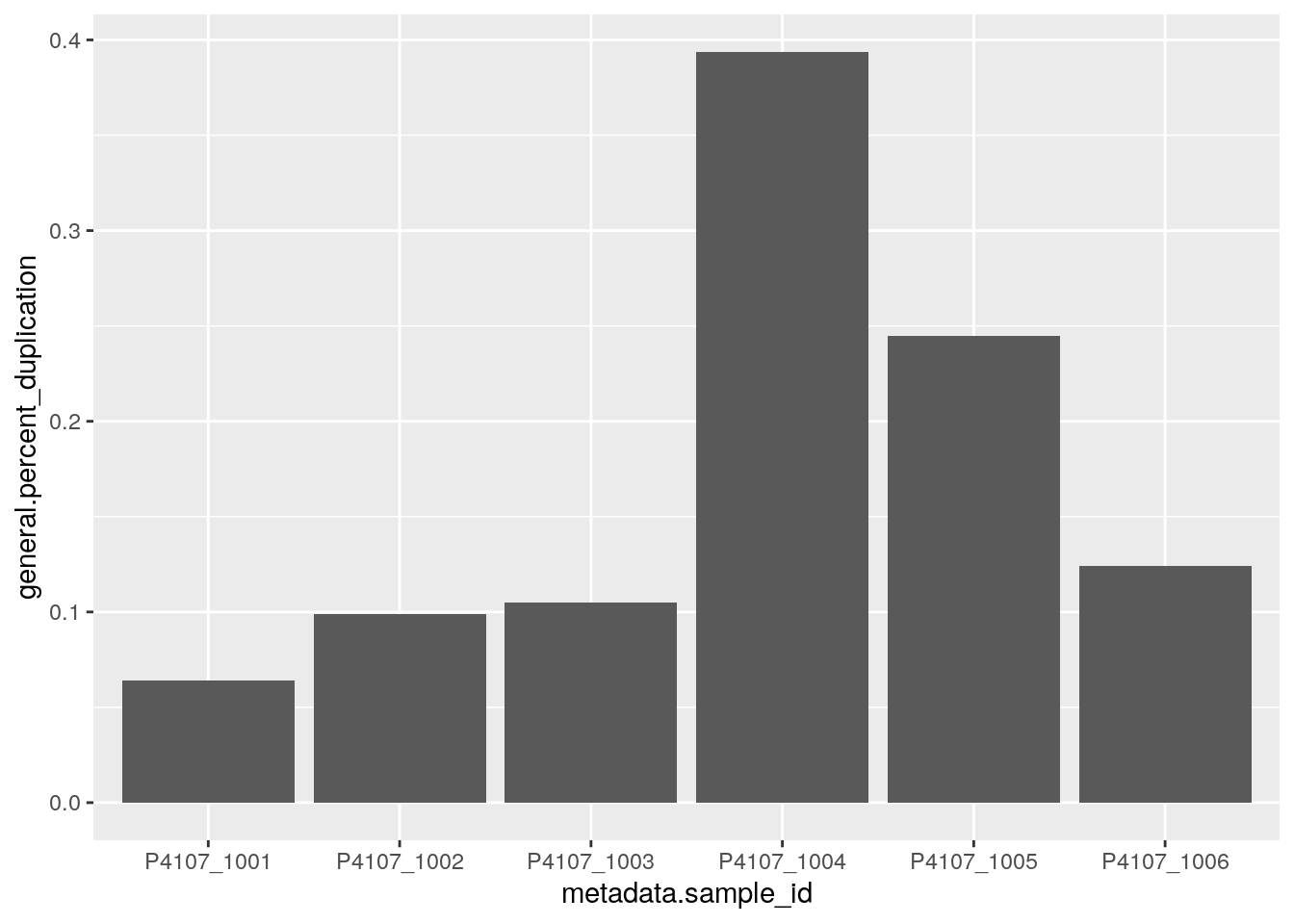## Test Data

In this example we’ll be using the WGS example report used in the MultiQC documentation. You can find view the report in your browser here, and find the associated multiqc_data.json file here. Feel free to download this file and use it to follow along with this vignette.

In the rest of the vignette we will use the multiqc_data_path variable to indicate the path to this multiqc_data.json file. Feel free to set this variable to the path to this file on your system.

## Setup

library(TidyMultiqc)

## Basic Usage

The main entry point to the TidyMultiqc package is the function load_multiqc. A basic invocation of this function looks like this:

df = load_multiqc(multiqc_data_path)
df

We’ve now generated a tibble (a kind of data frame), whose rows are samples in the QC report, and whose columns are QC data and metadata about these samples.

By default this function only returns the “general” statistics, which are the ones in the “General Statistics” table at the top of the MultiQC report. In TidyMultiqc, these statistics are all prefixed by general. We can also extract the “raw” statistics, which includes some fields normally hidden from the report. These statistics will have the prefix raw.<toolname>. where <toolname> is the QC tool used to calculate it.

load_multiqc(multiqc_data_path, sections = 'raw')

Often you won’t care about fields like raw.qualimap_bamqc_genome_results.bam_file, the path to the original BAM file, but ‘raw’ at least provides this option.

You can also combine both general and raw sections by passing in a longer vector:

df_both = load_multiqc(multiqc_data_path, sections = c('raw', 'general'))
ncol(df_both)
#>  388

That’s a lot of columns!

## Uses

This section will briefly talk about some downstream use-cases for this package.

### Plotting

One use for this data frame is creating QC plots. For example, to visualise the duplication rate per sample:

library(ggplot2)

ggplot(df, aes(x=metadata.sample_id, y=general.percent_duplication)) + geom_col()Of course, this is basically just replicating a plot already in the MultiQC report, but now we can customise it how we like!

### Hypothesis Testing

With all this data, we might also want to test a specific hypothesis! For example, we might want to test the hypothesis that the mean GC content is the same as the mean GC content in the human genome (41%). If we assume that GC content is normally distributed, we can do the following:

t.test(df$general.percent_gc, mu=41) #> #> One Sample t-test #> #> data: df$general.percent_gc
#> t = -1, df = 5, p-value = 0.3632
#> alternative hypothesis: true mean is not equal to 41
#> 95 percent confidence interval:
#>  40.40490 41.26176
#> sample estimates:
#> mean of x
#>  40.83333

It seems that we cannot reject this hypothesis, so these may well be human samples!

## Extracting Plot Data

It is occasionally useful to extract QC data from the MultiQC plots. For example, let’s say we want to calculate the median quality score of every base in each sample. Unfortunately, MultiQC provides no numerical summary statistic for the mean read quality, it only has mapping quality and pass/fails for the per-base sequence quality:

library(dplyr)
#>
#> Attaching package: 'dplyr'
#> The following objects are masked from 'package:stats':
#>
#>     filter, lag
#> The following objects are masked from 'package:base':
#>
#>     intersect, setdiff, setequal, union

df_both %>% select(contains('quality'))

However, our MultiQC report does have plots that contain this information. In particular, let’s look at the “Per Sequence Quality Scores” plot.

To extract this, we need to provide some new arguments to load_multiqc:

load_multiqc(
multiqc_data_path,
sections = 'plots',
plot_opts = list(
fastqc_per_sequence_quality_scores_plot = list(
extractor = extract_histogram,
summary = list(median=median),
prefix = "quality"
)
)
)

This looks pretty good! To walk through what we did here, we firstly specified the plots section. We also provided the plot_opts argument as a named list. The names of this list correspond to the plot names inside the MultiQC file (you can find them by looking in the report_plot_data section of the multiqc_data.json file). The values of this list are each a list of options for that plot. In this case, we used the following options:

• prefix = 'quality' means that we want to rename the plot to quality, rather than use its uglier default name of qualimap_coverage_histogram.
• extractor = extract_histogram means that this is a histogram plot (ie the y-axis are counts, and the x-axis are observations). Have a look at the reference manual for more types of extractor function.
• summary = list('median' = median) is a list of summary statistics to apply to the data we have extracted. In this case, we want to take the median, so we pass in the median function. The name corresponding to this function is the name that will end up in the final data frame.